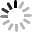## Basic Characteristics of NTC Thermistor

April 14, 2014

 Basic Characteristics of NTC Thermistor
 What is NTC Thermistor? NTC: Negative Temperature Coefficient Thermistor: Thermally Sensitive Resistor

Thermistor is a semiconducting ceramic resistor produced by sintering the materials at high temperature and made mainly from metal oxide. NTC thermistors are resistors with large negative temperature coefficient. Change in resistance of the NTC thermistors can be brought about either externally by a change in ambient temperature or internally by self-heating resulting from a current flowing through the device. At certain measure power, its resistance reduce rapidly while the temperature getting increase. Taking advantage of this characteristic, it can achieve the detecting and controlling temperature purpose.

 1. Zero-power resistance (RT)

The zero-power resistance is the value of a resistance when measured at a specified temperature, under conditions such that the change in resistance due to the internal generation of heat is negligible with respect to the total error of measurement.

 2、Rated zero-power resistance R25

The rated zero-power resistance is the nominal value at the standard temperature of 25℃ unless otherwise specified.

 3、B constant in Kelvin’s (K)

B constant is an index of the thermal sensitivity expressed by the formula:Resistance in ohms ( ) at temperature T1

Resistance in ohms ( ) at temperature T2

T1=2731.5k +( T1℃),  T2=2731.5k+ (T2℃)

 4、Dissipation factor(δ)

δ=ΔP/ΔT (mW/℃)

Dissipation factor (δ) is power in milliwatts required to raise thermistor temperature 1℃.Measured with thermistor suspended by its leads in a specified environment.

 5、Thermal time constant (τ)

Thermal time constant (τ) is the time required by a thermistor to change 63.2% of the difference between its initial and final temperature. Measured with thermistor suspended by its leads in specified environment.

τ=C/δ6、 Power rating

Rated power=heat dissipation factorδ × (maximum operating temperature－25℃)

 7、Resistance/temperature characteristic

The relationship between the zero-power resistance of a thermistor and its body temperature. The resistance law follow approximately the formula:R1 : Resistance value at absolute temperature T1 (K)
B : B constant T(K)=T(℃)+273.15